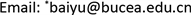Oldroyd-B流体，非稳态驻点流动，磁场，DPTEM-BF方法, Oldroyd-B Fluid Unsteady Stagnation-Point Flow Magnetic Field DPTEM-BF Method

Oldroyd-B流体，非稳态驻点流动，磁场，DPTEM-BF方法

Unsteady Stagnation-Point Flow of Oldroyd-B Fluid along a Stretching Sheet with Magnetic Field<sup> </sup>

Yu Bai1,2*, Qing Wang1,2, Yan Zhang1,2

1Beijing University of Civil Engineering and Architecture, Beijing

2Beijing Key Laboratory of Functional Materials for Building Structure and Environment Remediation, BeijingReceived: Oct. 19th, 2020; accepted: Dec. 28th, 2020; published: Jan. 5th, 2021ABSTRACT

This paper examines the influence of magnetic field on unsteady stagnation-point flow of Oldroyd-B fluid towards a stretching sheet. Suitable similarity transformations are put into use to yield the ordinary differential equation, which are dealt with double-parameter transformation expansion method with base function method (DPTEM-BF). Impacts of various physical parameters on the velocity field are explored via graphs. It is noteworthy that as relaxation time parameter enlarges, the viscous force of fluid increases, which causes larger resistance to fluid flow. The increase of retardation time parameter increases both the velocity and momentum boundary layer thickness. Larger magnetic parameter corresponds to the larger Lorentz force, which impedes fluid flow and thus slows it down.

Keywords:Oldroyd-B Fluid, Unsteady Stagnation-Point Flow, Magnetic Field, DPTEM-BF Method1. 引言

2. 数学模型

Oldroyd-B流体的非稳态驻点流动控制方程为：

∂ u ∂ x + ∂ v ∂ y = 0 (1)

∂ u ∂ t + u ∂ u ∂ x + v ∂ u ∂ y = − λ 1 ( ∂ 2 u ∂ t 2 + 2 u ∂ 2 u ∂ x ∂ t + 2 v ∂ 2 u ∂ y ∂ t + u 2 ∂ 2 u ∂ x 2 + 2 u v ∂ 2 u ∂ x ∂ y + v 2 ∂ 2 u ∂ y 2 )     + ν ∂ 2 u ∂ y 2 + λ 2 ν ( ∂ 3 u ∂ y 2 ∂ t + v ∂ 3 u ∂ y 3 + u ∂ 3 u ∂ x ∂ y 2 − ∂ u ∂ x ∂ 2 u ∂ y 2 − ∂ u ∂ y ∂ 2 v ∂ y 2 )     + ∂ u e ∂ t + u e ∂ u e ∂ x + λ 1 u e 2 ∂ 2 u e ∂ x 2 − σ B 0 2 ρ [ u − u e + λ 1 ( ∂ u ∂ t + v ∂ u ∂ y − ∂ u e ∂ t ) ] (2)

u = b x ( 1 − a t ) f ′ ( η ) , v = − ν b ( 1 − a t ) f ( η ) , η = b ν ( 1 − a t ) y , ψ = ν b ( 1 − a t ) x f ( η ) (5)

S ( f ′ + 1 2 f ″ η ) + ( f ′ ) 2 − f f ″ + β 1 { S 2 ( 2 f ′ + 7 4 f ″ η + 1 4 f ‴ η 2 ) + 2 S [ ( f ′ ) 2 + 1 2 f ′ f ″ η ]   − S ( 3 f f ″ + f f ‴ η ) − 2 f f ′ f ″ + f 2 f ‴ } − f ‴ − β 2 [ S ( 2 f ‴ + 1 2 f i v η ) − f f i v + ( f ″ ) 2 ]   − A S − A 2 + M { ( f ′ − A ) + β 1 [ S ( f ′ + 1 2 f ″ η ) − f f ″ − S A ] } (6)

3. DPTEM-BF方法求解

f ( η ) = ε 4 F ( ξ ) + f ( 0 ) + f ′ ( 0 ) η + f ″ ( 0 ) 2 ! η 2 + f ‴ ( 0 ) 3 ! η 3 ， ξ = ε − 1 η (9)

F ( 0 ) = 0 ， F ′ ( 0 ) = 0 ， F ″ ( 0 ) = 0 ， F ‴ ( 0 ) = 0 (10)

F ( ξ ) = F 0 ( ξ ) + ∑ i = 1 ∞ F i ( ξ ) ε i (11)

F i ( 0 ) = 0 ， F ′ i ( 0 ) = 0 ， F i ′ ​ ′ ( 0 ) = 0 ， F i ′ ​ ′ ​ ′ ( 0 ) = 0 ， i = 0 , 1 , 2 , 3, ⋯ (12)

f ( η ) ≈ f N 1 , N 2 ( η ) = f 0 , 0 ( η ) + ∑ j = 3 N 1 ∑ i = 1 N 2 a i j f i , j ( η ) = f 0 , 0 ( η ) + ∑ j = 3 N 1 ∑ i = 1 N 2 a i j η j e i a 0 η (13)

f 0 , 0 ( η ) = m 1 + s η − m 1 e a 0 η + ( 1 − s + a 0 m 1 ) η e a 0 η + m 2 η 2 e a 0 η (14)

4. 结果与讨论

Comparison of − f ″ ( 0 ) with different values of S while β 1 = β 2 = A = M =
SSharidan et al. Chamkha et al. Mukhopadhyay et al. Present results
0.81.2610421.2615121.2614791.261826
1.21.3777221.3780521.3778501.377496

5. 总结